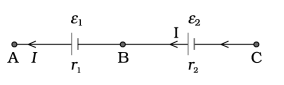# Qn) a) State whether the following statement is correct. ‘‘If the current through the cell is from its positive to negative, against the direction of emf, then the potential drop across the internal resistance aids the emf of the cell.’’ b) You are given two cells. Group them so that they give more voltage. Arrive at the expression for effective emf and internal resistance.

a) True. Internal resistance and lost volt always opposes current flow through the cell.
b)Consider two cells in series e1 , e2 are the emf’s of the two cells and r1 , r2 their internal resistances,

respectively.Let V (A), V (B), V (C) be the potentials at points A, B and C shown in Fig. Then V (A)
– V (B) is the potential difference between the positive and negative terminals of the
first cell.
V AB ≡ V (A) − V (B) = ε 1 − I r 1

Similarly,
V BC ≡ V (B) − V (C) = ε2 − I r2
Hence, the potential difference between the terminals A and C of the combination is
V AC ≡ V (A) − V (C ) = [ V ( A ) − V ( B ) ]+ [ V ( B ) − V ( C ) ]
= ( ε1 + ε2 ) − I ( r1 + r2 )
V AC = εeq – I req
Comparing the last two equations, we get
ε eq = ε1 + ε2   and req = r1 + r2